# Magnetic Field of Current

The magnetic field lines around a long wire which carries an electric current form concentric circles around the wire. The direction of the magnetic field is perpendicular to the wire and is in the direction the fingers of your right hand would curl if you wrapped them around the wire with your thumb in the direction of the current.Calculation Bend current into loop
Index

Magnetic field concepts

Currents as magnetic sources

 HyperPhysics***** Electricity and Magnetism R Nave
Go Back

# Magnetic Field of Current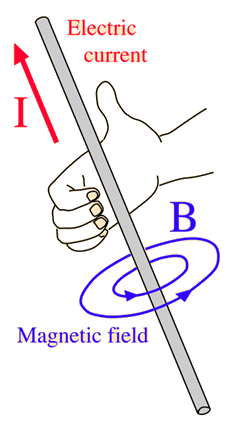The magnetic field of an infinitely long straight wire can be obtained by applying Ampere's law. The expression for the magnetic field is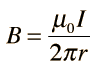Show
 For a current I = Amperes and
 the magnetic field is B = Tesla = Gauss B = x10^Tesla = x10^ Gauss.

The earth's magnetic field is about 0.5 gauss. The permeability of free space is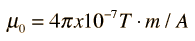Magnetic force between wires
Index

Magnetic field concepts

Currents as magnetic sources

 HyperPhysics***** Electricity and Magnetism R Nave
Go Back

# Magnetic Field of Current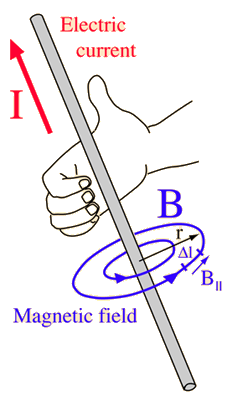The magnetic field of an infinitely long straight wire can be obtained by applying Ampere's law. Ampere's law takes the form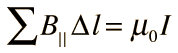and for a circular path centered on the wire, the magnetic field is everywhere parallel to the path. The summation then becomes just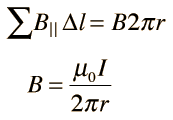The constant μ0 is the permeability of free space.

### Calculation

Index

Magnetic field concepts

Currents as magnetic sources

 HyperPhysics***** Electricity and Magnetism R Nave
Go Back